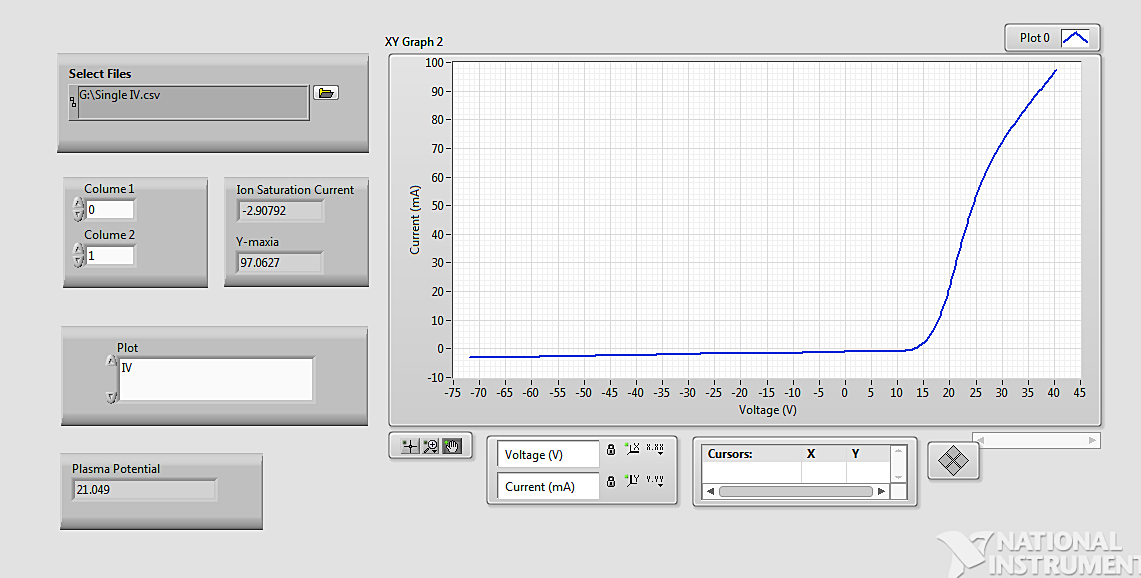# LabVIEW

cancel
Showing results for
Did you mean:

## how to find out the x intercept

Hi,

suppose I have X and Y data points and plotted on XY graph. Now I want to find out the value of X intercept i.e. value of X at which Y=0. Suppose in my data I don't have X value where Y=0. Should I have to interpolate my Y axis to find out the X intercept and if it is so how to do that.

Message 1 of 5
(2,060 Views)

## Re: how to find out the x intercept

What kind of function is it?

In general case you can use Mathematics -> Scripts & Formulas -> Zeros -> Find all zeros function.

As it needs values between the points, you will need to interpolate.

In simple case (monotonic function) you can use Threshold 1D array for Y to get fractional index of your zero, then Interpolate 1D array to get X.

Message 2 of 5
(2,046 Views)

## Re: how to find out the x intercept

@bdcpp08 wrote:

suppose I have X and Y data points and plotted on XY graph. Now I want to find out the value of X intercept i.e. value of X at which Y=0. Suppose in my data I don't have X value where Y=0. Should I have to interpolate my Y axis to find out the X intercept and if it is so how to do that.

If you have data on both sizes of zero, you need to interpolate. If the data does not coss zero, you need to extrapolate.

This is a trivial problem for clean data, but can be complicated in the presence of noise (it might cross several times over a very narrow range).

How does the data look like? How many times does it cross zero? Is it e.g. nondescending? How much noise do you have? Can it be fit with a low order polynomial, for example?

Please attach a VI containing typical data.LabVIEW Champion. It all comes together inGCentralWhat does "Engineering Redefined" mean??
Message 3 of 5
(2,030 Views)

## Re: how to find out the x intercept

Hi altenbach,

here I am sending the snapshot of the front panel of my program along with the data and the vi.Message 4 of 5
(1,979 Views)

## Re: how to find out the x intercept

Try something like this....(again, this assumes that the Y array is nondescending. If you have noise, you need better tools. There are altrernative versions that operate on the xy cluster array directly)LabVIEW Champion. It all comes together inGCentralWhat does "Engineering Redefined" mean??
Message 5 of 5
(1,953 Views)